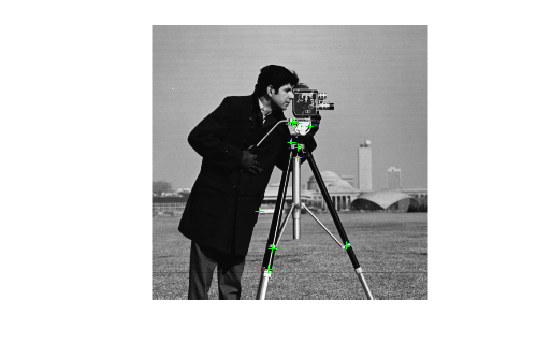# cornerPoints

Object for storing corner points

## Description

This object stores information about feature points detected from a 2-D grayscale image.

## Creation

### Syntax

``points = cornerPoints(location)``
``points = cornerPoints(location,Name,Value)``

### Description

example

````points = cornerPoints(location)` constructs a `cornerPoints` object from an M-by-2 array [x y] of location coordinates. ```
````points = cornerPoints(location,Name,Value)` sets properties using one or more name-value pairs. Enclose each property name in quotes. For example, `points = cornerPoints('Metric',0.0)````

### Input Arguments

expand all

Location of points, specified as an M-by-2 array of [x y] coordinates.

## Properties

expand all

Location of keypoints, specified as an M-by-2 matrix. Each row is of the form [x y] and represents the location of a keypoint. M is the number of keypoints. You cannot set this property, use the `location` input argument instead.

Number of points held by the corner points object, specified as a numeric value.

Strength of detected feature, specified as a numeric value. The algorithm uses a determinant of an approximated Hessian.

## Object Functions

 `plot` Plot points `isempty` Determine if points object is empty `length` Number of stored points `selectStrongest` Select points with strongest metrics `size` Return size of points object `selectUniform` Select uniformly distributed subset of feature points `gather` Retrieve cornerPoints from the GPU

## Examples

collapse all

`I = imread('cameraman.tif');`

Detect feature points.

`points = detectHarrisFeatures(I);`

Display the ten strongest points.

```strongest = selectStrongest(points,10); imshow(I) hold on plot(strongest)```Display the (x,y) coordinates of the strongest points.

`strongest.Location`
```ans = 10x2 single matrix 112.4516 208.4412 108.6510 228.1681 136.6969 114.7962 181.4160 205.9876 135.5823 123.4529 100.4951 174.3253 146.7581 94.7393 135.2899 92.6485 129.8439 110.0350 130.5716 91.0424 ```

Create a checkerboard image.

`I = checkerboard(50,2,2);`

Load the locations of corner points.

```location = [51 51 51 100 100 100 151 151 151; ... 50 100 150 50 101 150 50 100 150]';```

Save the points in a `cornerPoints` object.

`points = cornerPoints(location);`

Display the points on the checkerboard.

```imshow(I) hold on plot(points)```## Tips

Although `cornerPoints` may hold many points, it is a scalar object. Therefore, `numel`(`cornerPoints`) always returns `1`. This value may differ from `length`(`cornerPoints`), which returns the true number of points held by the object.

## Version History

Introduced in R2012a